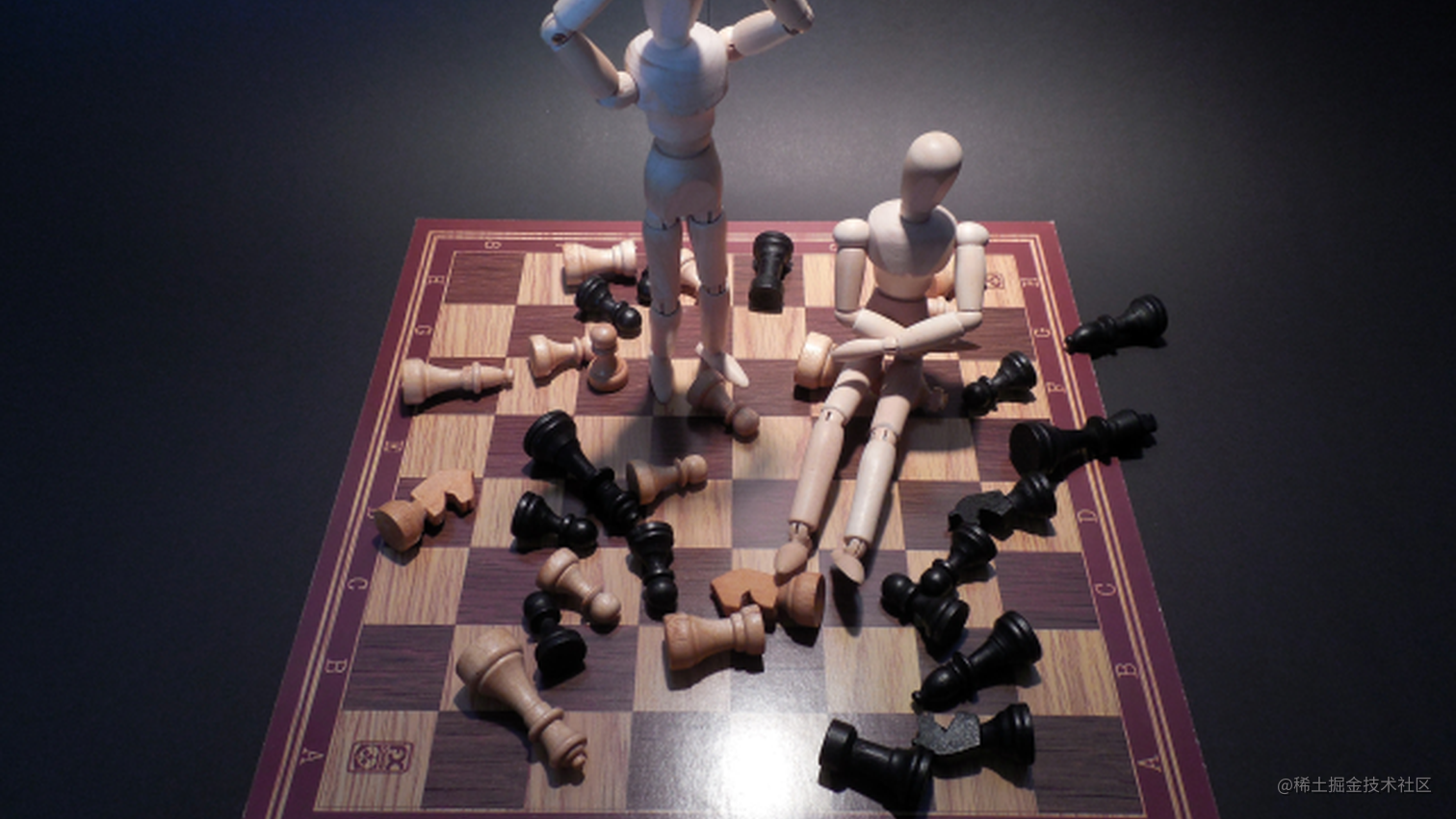# 你真的懂 == 和 equals 的区别吗？## 1、== 解读

• 基本类型：比较的是值是否相同；
• 引用类型：比较的是引用是否相同；

``````String x = "string";
String y = "string";
String z = new String("string");
System.out.println(x==y); // true
System.out.println(x==z); // false
System.out.println(x.equals(y)); // true
System.out.println(x.equals(z)); // true

## 2、equals 解读

equals 本质上就是 ==，只不过 String 和 Integer 等重写了 equals 方法，把它变成了值比较。看下面的代码就明白了。

``````class Cat {
public Cat(String name) {
this.name = name;
}

private String name;

public String getName() {
return name;
}

public void setName(String name) {
this.name = name;
}
}

Cat c1 = new Cat("王磊");
Cat c2 = new Cat("王磊");
System.out.println(c1.equals(c2)); // false

``````public boolean equals(Object obj) {
return (this == obj);
}

``````String s1 = new String("老王");
String s2 = new String("老王");
System.out.println(s1.equals(s2)); // true

``````public boolean equals(Object anObject) {
if (this == anObject) {
return true;
}
if (anObject instanceof String) {
String anotherString = (String)anObject;
int n = value.length;
if (n == anotherString.value.length) {
char v1[] = value;
char v2[] = anotherString.value;
int i = 0;
while (n-- != 0) {
if (v1[i] != v2[i])
return false;
i++;
}
return true;
}
}
return false;
}